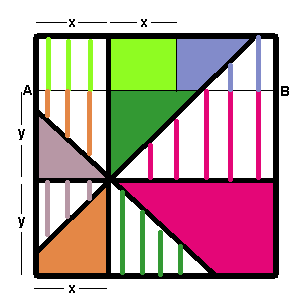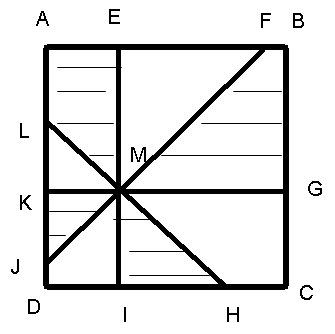#### You may also like### At a Glance

The area of a regular pentagon looks about twice as a big as the pentangle star drawn within it. Is it?### Six Discs

Six circular discs are packed in different-shaped boxes so that the discs touch their neighbours and the sides of the box. Can you put the boxes in order according to the areas of their bases?### Equilateral Areas

ABC and DEF are equilateral triangles of side 3 and 4 respectively. Construct an equilateral triangle whose area is the sum of the area of ABC and DEF.

# Square Pizza

##### Age 14 to 16Challenge Level

Two solutions offered by Andrei of School 205, Bucharest. Many thanks Andrei.

I solved the problem in two ways:

## Method 1.I find from reasons of symmetry the equality of areas

Without loss of generality, I suppose x < l/2, and y < l/2.

I start from the observation that there are from the beginning two congruent triangles, the one coloured in purple, and the other with purple lines.

I look now to the trapezium coloured continuously in red, and I try to find another one, congruent with this one. For this, I trace a line AB parallel to the base of the square, at 2y. It is drawn in the figure.

I see the equality of the areas of the two trapeziums the one in continuous red, the other with red lines.

The line drawn determines another 4 trapeziums, with equal areas two by two, and that could be identified from the colour: green and orange.

Now, I remained with all the area above the line AB just drawn. To find equal areas, I drew another line, parallel with the vertical side of the square, at 2x.

The equal areas found are again with the same colour, line and continuous.

Now, the part of the pizza with lines has the same area with the part of the pizza with continuous colours, and it is easy to join the parts that have been determined by the new lines.

## Method 2.

I calculated the areas of all figures (triangles and rectangular trapeziums) delimitated by the sides of the pizza and by the cuts.

I noted all the points from the figure (see chart). Now, let the length of DI be x and the length of DK be y; let the side of the square be 1 unit.A LMK = x 2 /2
A KJM = x 2 /2
A DJMI = xy - x 2 /2
A HCGM = 1y - xy - y 2 /2
A MFBG = (1 2 - y 2 )/2 - 1x + xy
A LAEM = 1x - xy - x 2 /2
A EMF = 1 2 /2 - 1y + y 2 /2

Now, look what areas to add to obtain 1 2 /2.

First I take triangle EMF, whose area begins with 1 2 /2. Because 1y is subtracted from 1 2 /2, I must look for an area that contains (+ 1y). The only area containing such a number is MHCG. I write the obtained area up to now:

AEMF + AHCGM = 1 2 /2 - 1y + y 2 /2 + 1y - xy - y 2 /2 = 1 2 /2 - xy

Now, I must add something containing (+xy). I add LKM and DJMI, obtaining 1 2 /2. For the remaining areas the sum is naturally 1 2 /2.

So, I added the areas in the following manner:

ALKM + ADJMI + AMHCG + AEMF and
AKJM + AMIH + AMFBG + ALAEM.

These two kind of pieces are marked differently on the figure.